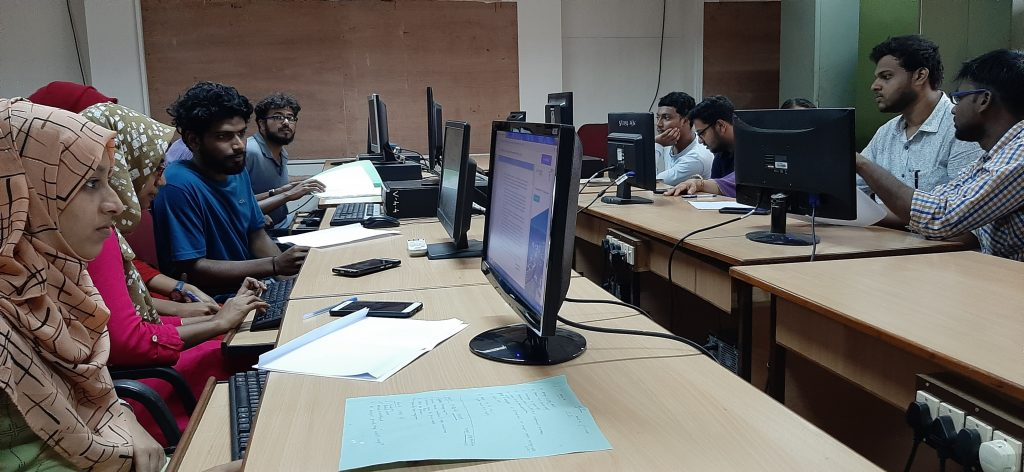# Digital Signal Processing LabLab in charge:

• Faculty: Dr. Sivakumar R
• Technical Staff: Ms. Kala S

Details of Labs conducted:

• ECL333 – DIGITAL SIGNAL PROCESSING LAB – S5 ECE
• EST102 – PROGRAMMING IN C – S2 AEI, ECE

### ECL333 – DIGITAL SIGNAL PROCESSING LAB

This course aims to:

• Make the student do real time DSP computing. Dedicated DSP hardware (such as TI or Analog Devices development/evaluation boards) will be used for realization.

LIST OF EXPERIMENTS:

1. Simulation of Signals
2. Verification of the Properties of DFT
3. Familarization of DSP Hardware
4. Linear convolution
5. FFT of signals using DIT
6. FFT of signals using DIF
7. IFFT with FFT
8. FIR low pass filter
9. IIR filter design
10. Overlap Save Block Convolution

### EST102 – PROGRAMMING IN C

LIST OF EXPERIMENTS:

1. Familiarization of Hardware Components of a Computer
2. Familiarization of Linux environment – How to do Programming in C with Linux
3. Familiarization of console I/O and operators in C
4. Read 3 integer values and find the largest amoung them
5. Read a Natural Number and check whether the number is prime or not
6. Read a Natural Number and check whether the number is Armstrong or not
7. Read n integers, store them in an array and find their sum and average
8. Read n integers, store them in an array and search for an element in the array using an algorithm for Linear Search
9. Read n integers, store them in an array and sort the elements in the array using Bubble Sort algorithm
10. Read a string (word), store it in an array and check whether it is a palindrome word or not.
11. Read two strings (each one ending with a \$ symbol), store them in arrays and concatenate them without using library functions.
12. Read a string (ending with a \$ symbol), store it in an array and count the number of vowels, consonants and spaces in it.
13. Read two input each representing the distances between two points in the Euclidean space, store these in structure variables and add the two distance values
14. Using structure,read and print data of n employees (Name,Employee Id and Salary)
15. Declare a union containing 5 string variables (Name, House Name, City Name, State and Pin code) each with a length of C_SIZE (user defined constant). Then, read and display the address of a person using a variable of the union.
16. Find the factorial of a given Natural Number n using recursive and non recursive functions
17. Read a string (word), store it in an array and obtain its reverse by using a user defined function.
18. Write a menu driven program for performing matrix addition, multiplication and finding the transpose. Use functions to (i) read a matrix, (ii) find the sum of two matrices, (iii) find the product of two matrices, (i) find the transpose of a matrix and (v) display a matrix.
19. Do the following using pointers